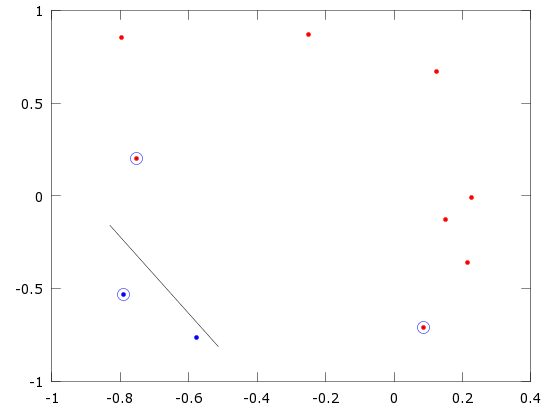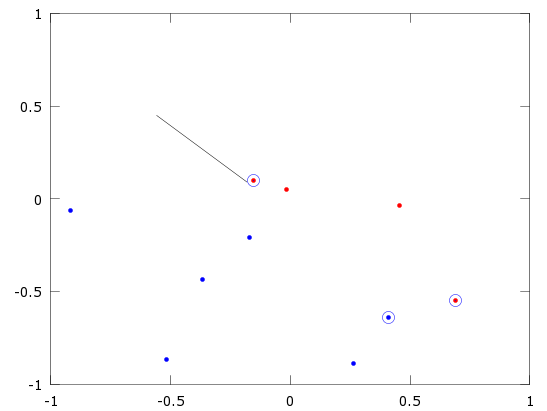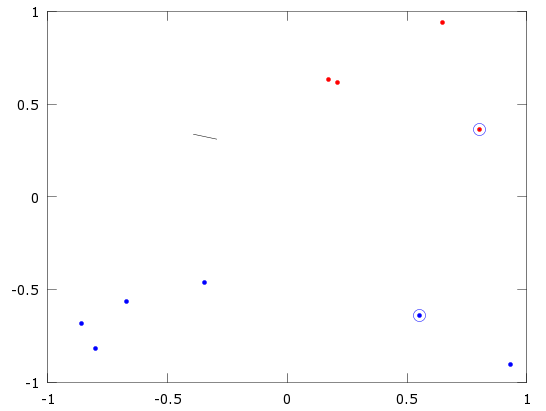#18
 invisSenior Member Join Date: Jul 2012 Posts: 50Re: Quadratic programming

Hmm, my SV looks pretty strangeCode:
```    options = optimset("MaxIter", 400);
H = (Y*Y') .* (X*X');
A = Y';
q=-1*ones(n,1);
b=0;
lb=zeros(n,1);
ub=10^10*ones(n,1);
alpha0 = qp ([], H+(eye(n)*10^-15), q, A, b, lb, ub, options);
alpha = qp (alpha0, H, q, A, b, lb, ub, options);```
After this I have Alpha vector size of n. Next check what of alphas bigger then threshold (mine is 10^-5). If Alpha bigger then threshold this mean that X[2,:] (X is my n*2 matrix with points) is one of support vectors
Ok, look at this vectors (small black line is my):Some of them pretty good, I place here only strange. Any ideas about behavior of this support vectors ?

Or it is a part of homework ? Because I check with n=100 and vectors looks better, but some time some of them still strange